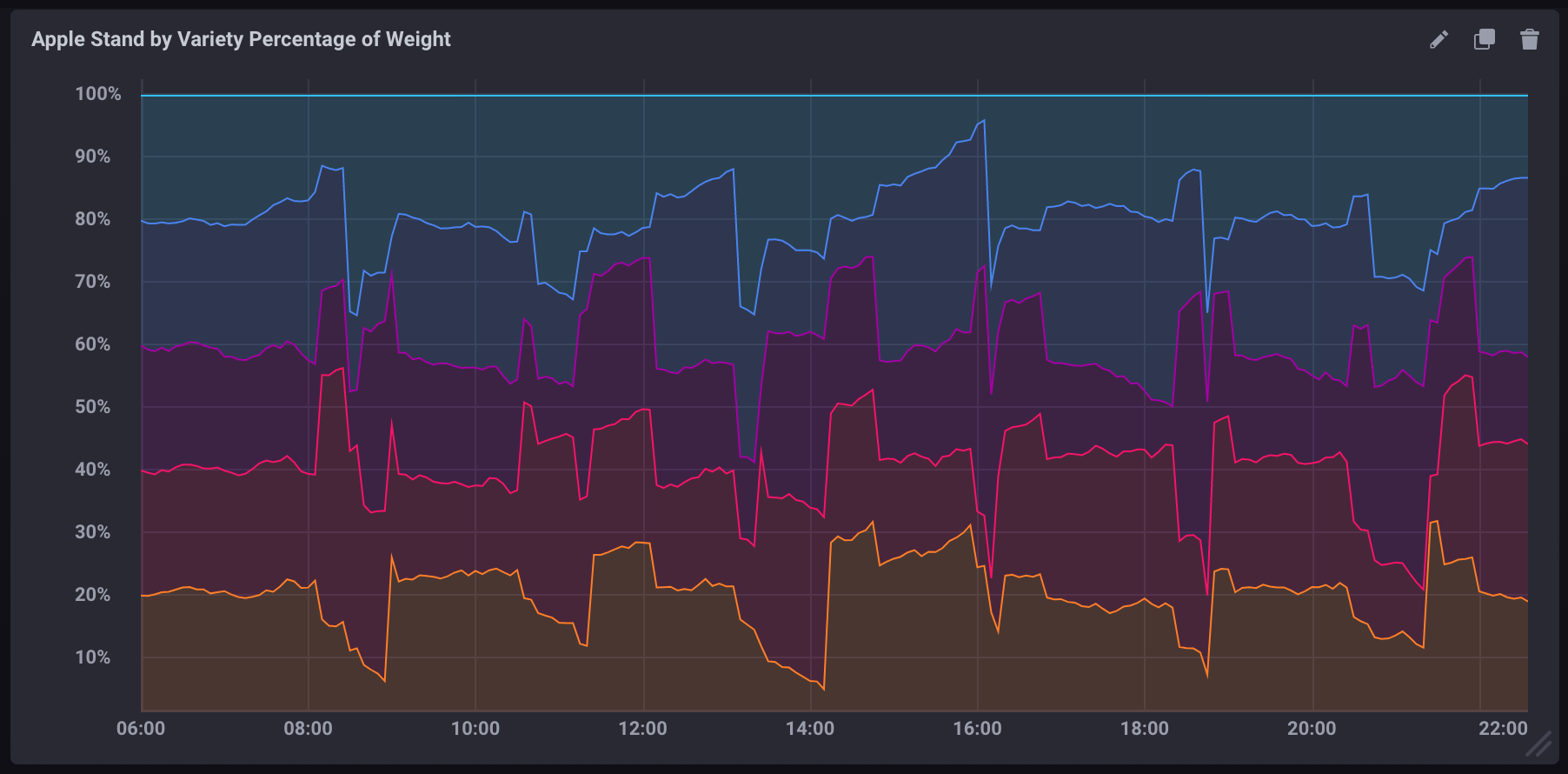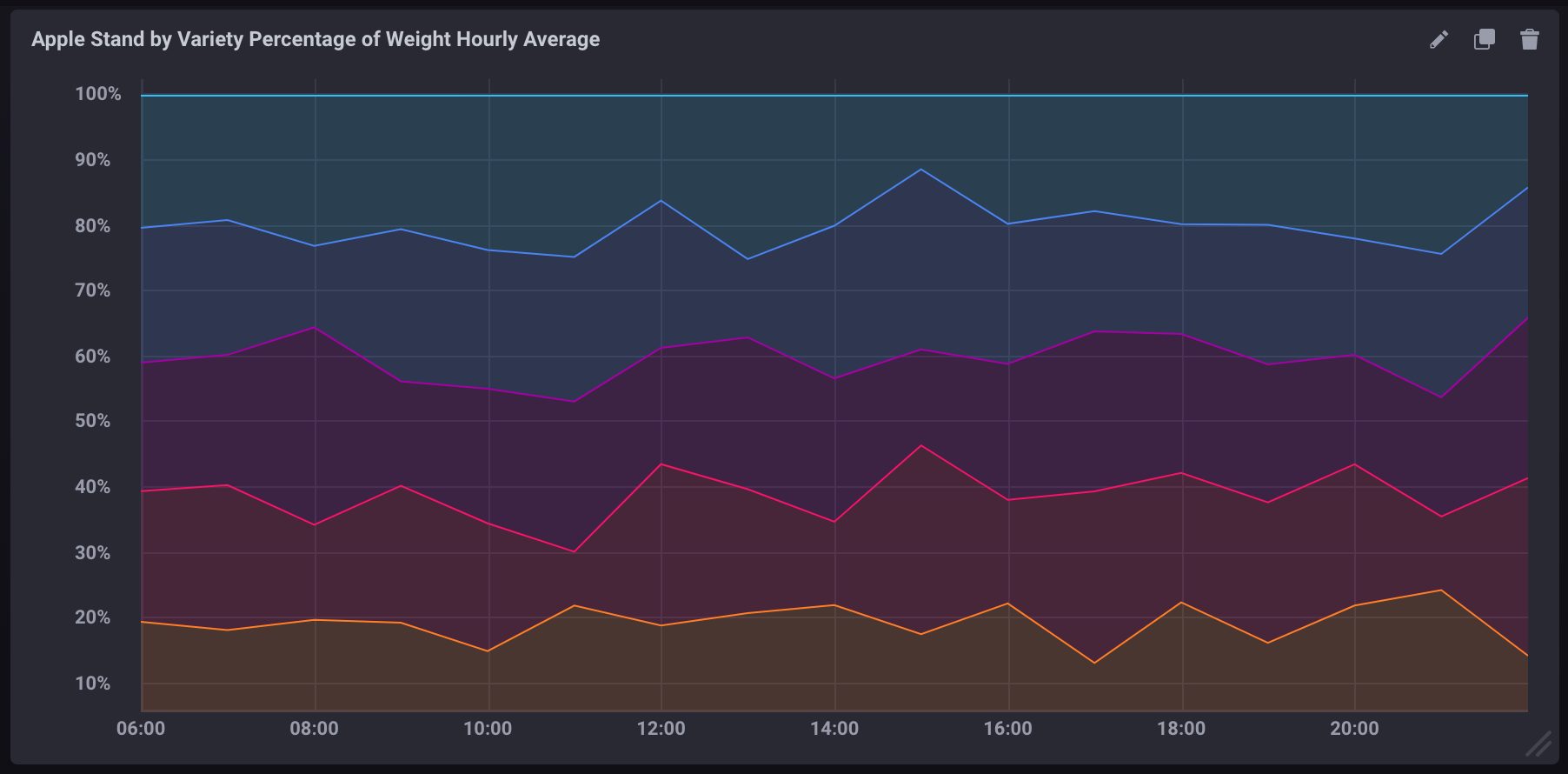Search

计算查询中的百分比

InfluxQL允许您计算简单的数学方程式，这使得使用measurement中的两个field字段计算百分比非常简单。然而，有一些注意事项不能忽略。

查询中的基本计算

SELECT语句支持使用基本的数学运算符，例如，+-/*()等等。

1. -- 两个field key相加
2. SELECT field_key1 + field_key2 AS "field_key_sum" FROM "measurement_name" WHERE time < now() - 15m
3. -- 两个field key相减
4. SELECT field_key1 - field_key2 AS "field_key_difference" FROM "measurement_name" WHERE time < now() - 15m
5. -- 分组计算并将它们连接起来
6. SELECT (field_key1 + field_key2) - (field_key3 + field_key4) AS "some_calculation" FROM "measurement_name" WHERE time < now() - 15m

计算查询中的百分比

1. SELECT (field_key1 / field_key2) * 100 AS "calculated_percentage" FROM "measurement_name" WHERE time < now() - 15m

使用聚合函数计算百分比

1. SELECT (sum(field_key1) / sum(field_key2)) * 100 AS "calculated_percentage" FROM "measurement_name" WHERE time < now() - 15m GROUP BY time(1m)

示例

示例数据

1. 下载示例数据
2. 导入示例数据：

1. influx -ssl -username <账号名称> -password <密码> -host <网络地址> -port 3242 -import -path=path/to/apple_stand.txt -database=apple_stand

计算每个品种占总重量的百分比

1. SELECT
2. ("braeburn"/total_weight)*100,
3. ("granny_smith"/total_weight)*100,
4. ("golden_delicious"/total_weight)*100,
5. ("fuji"/total_weight)*100,
6. ("gala"/total_weight)*100
7. FROM "apple_stand"."autogen"."variety"计算每个品种的聚合百分比

1. SELECT
2. (mean("braeburn")/mean(total_weight))*100,
3. (mean("granny_smith")/mean(total_weight))*100,
4. (mean("golden_delicious")/mean(total_weight))*100,
5. (mean("fuji")/mean(total_weight))*100,
6. (mean("gala")/mean(total_weight))*100
7. FROM "apple_stand"."autogen"."variety"
8. WHERE time >= '2018-06-18T12:00:00Z' AND time <= '2018-06-19T04:35:00Z'
9. GROUP BY time(1h)

• 它使用聚合函数（mean()）来提取所有数据
• 它包含一个GROUP BY time()子句，将数据按一小时聚合
• 它包含一个明确的时间区间，如果没有它，聚合函数是非常耗费资源的InfluxDB® is a trademark registered by InfluxData, which is not affiliated with, and does not endorse, TSDB for InfluxDB®.